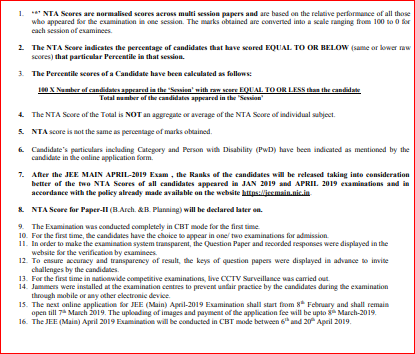# How to calculate Rank from JEE percentile? JEE Mains ResultHow to calculate Rank from JEE percentile?: National Testing Agency (NTA) has declared the result of Jee Main January 2019 on 19 January 2019 in online mode. The announced result is for the first JEE Main 2019 which was conducted by NTA between January 8 to 12 in two shifts per day across 258 cities in the country and abroad. A total number of 9, 29,198 candidates were registered for Paper I (B.E. /B. Tech. This year, NTA has released percentile score instead of Raw Score. NTA will release JEE Main 2019 All India Ranks (AIR) or Ranklist once the April exam session of the exam concludes. Now, the question is how the aspirants will calculate rank from their respective Percentile. Here, we have compiled all the information needed to know about the normalisation process set up by NTA for JEE Main 2019 scoresIt has been recorded that 15 candidates recording a 100 NTA score across India. According to sources, Ranks would be declared after the Second Session of JEE to be held on 06-20 April 2019.

## What is JEE Main Percentile?

First and foremost, one needs to know what is percentile of the result. As we are familiar with the JEE Main total marks which are 360 Marks. Similarly, let us suppose the JEE Main topper got 345 marks out of 360. Now, the topper marks will be considered as 100 percentile. The question is how to calculate Rank from Percentile?

So, the total number of candidates who appeared for JEE Main 2019 January exam is around 8.74 lakhs. The exam was conducted on four days in two slots (4 days x 2 slots = 8 sessions in total). So, approximately 1.09 lakh students took the exam in each session. Note that the JEE Main 2019 results/scorecard mentions only the NTA score for each subject and not the subject-wise marks secured by candidates. The percentile score displayed against each of the subjects and the overall percentile score is calculated up to 7 decimals using a pre-determined formula.

## How to calculate JEE Main 2019 rank based on NTA Percentile score?

So, to convert your JEE Main Percentile into a Rank, you need the following figures:

2. Total number of students who have appeared for the exam
3. Percentage of students below or equal to your marks
4. Percentage of students above your marks
5. Number of students above your marks

While the data for the first two aspects are easily available, the figures for the other three data points is difficult to find. To calculate these, here’s what you need to do.

Let’s suppose:

 Your NTA score = P Total Number of Students = N Percentage of students below or equal to your marks = P   Example: if you have secured a 70 percentile in JEE Main, which means that 70% of the candidates who appeared for JEE Main are below or at the same level as you are Percentage of students above your marks = 100 – P Number of students above your marks ((100 – P)/100)*N

## Formula to calculate JEE Main 2019 Rank from Percentile Score

Now, the formula to calculate JEE Main 2019 Rank from Percentile Score is: ((100 – P)/100)*N + 1

The rank generated will be the probable All India Rank you can expect to secure in JEE Main 2019. The actual rank might differ owing to different factors.Let us see an example here:

Two Candidates X & Y both appeared for the JEE Main 2019 in January and April as well. If candidate X gets 94 percentile in January and 65 percentile in April. Whereas, the student Y gets 80 percentile in January and 98 percentile in April.

Similarly, in this case, Candidate Y have higher ranks as compared to candidate X because JEE Main 2019 final result will be based upon best of two i.e., Candidate X January marks and Candidate Y April marks will be taken to mark rank card. I hope you are cleared with the concept of JEE Main marking system and moreover, got the answer to your question.

 January April CANDIDATE X 94 Percentile 65 Percentile CANDIDATE Y 80 Percentile 98 Percentile

## Key Points to Remember about JEE Main Percentile

• Percentile is not the same as the percentage.
• JEE Main merit list will be prepared on the basis of percentile scores and not on the raw marks secured by aspirants in the exam.
• Percentile = (No. of students in your shift with a score greater than or equal to your score) / (total number of students in your shift)
• For the 8 students with percentile 90 in Shift 1 and percentile 90 in Shift 2 and so onâ€¦ the tie-breaker will be on the basis of
• Percentile in Math followed by
• Percentile in Physics followed by
• Percentile in Chem followed by
• Age (older wins)
• What is the translation of percentile to Rank?
• Roughly 10 lakh students have taken the JEE Mains
• This means that each shift has 1 lakh 25 thousand, candidates.
• So a “qualification” percentile is around 90.
• NIT selection will be at around 96.
• You are on track for an IIT selection at 99 percentile and above
• Of course – this JEE is best of 2. So there is a chance to improve next time.

Stay tuned “How to calculate Rank from JEE percentile?” Here.

The post How to calculate Rank from JEE percentile? JEE Mains Result appeared first on Engineering Exams.